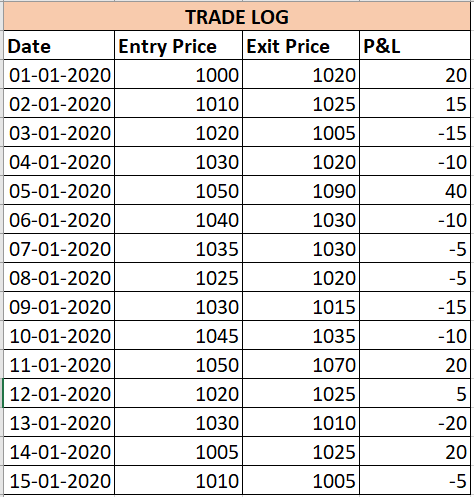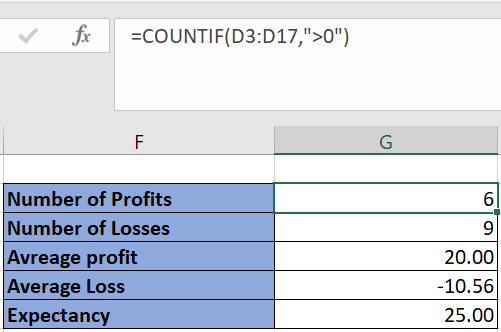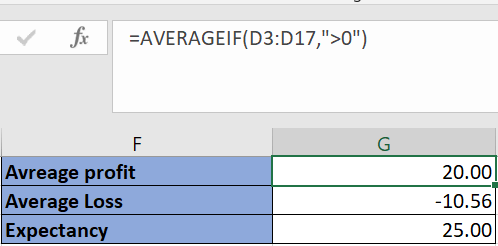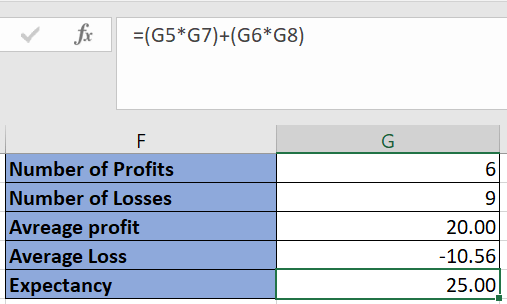# How to Calculate Expectancy in an Excel Sheet?

Expectancy is an important metric that tells you how much profit each trade is going to produce. It can be both a positive or negative number. A positive expectancy signifies a net profitable trading system. In this post, we’ll learn how to calculate expectancy in an excel sheet.

## Expectancy Calculation

Below is the mathematical formula used to calculate expectancy:

Expectancy = (Number of Profits * Average Profit Value) + (Number of Loss * Average Loss Value)

For accurate estimation of expectancy, it is recommended that you have a record for at least 15 trades.

From the formula, you can understand that expectancy would be positive if either of “Number of Profits” or “Average Profit Value” is a high number.

In practical scenarios, it is difficult to ensure that every trade is profitable, so traders must aim for higher average profit to increase expectancy. This is analogous to the risk-reward ratio.

Most of the modern backtesting platforms already have expectancy as a metric in their backtesting report.

## Calculate Expectancy in an Excel Sheet

In order to calculate expectancy, the first prerequisite is to have some kind of trade log or backtest log. This trade log contains date wise P&L for all the trades that your system has generated.

See below image for example:Next, based on the trade log you’ll have to calculate the number of profits and losses.

Use the “COUNTIF” function on your P&L column to do so. The second argument of countif would be “>0” when you are calculating number of profits, and it will be “<0” when you are calculating number of losses.Similarly, average profit and loss are calculated using the “AVERAGEIF” function on the P&L column.Finally, expectancy is calculated by adding the product of number of profits and average profit and number of loss and average loss.It is a positive value in the above case and signifies that each trade can give typically produce a profit of 25.